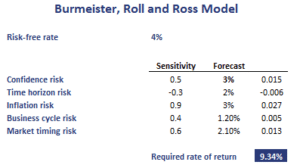# Burmeister, Roll, and Ross model

The Burmeister, Roll, and Ross model or Burmeister, Ibbotson, Roll and Ross model (BIRR) model is a macroeconomic multifactor model. The model uses factors associated with economic variables that should be expected to influence cash flows and/or discount rates. In particular, the model incorporates 5 factors; confidence risk, time horizon risk, inflation risk, business cycle risk, and market timing risk. The BIRR model was proposed in 1994 and is widely used by analysts every since.

On this page, we discuss the Burmeister, Roll, and Ross model formula, the definition of the underlying factors, and a simple numerical example that uses estimates on the factors to calculate the required return. The spreadsheet example is available for download at the bottom of the page.

## Burmeister, Roll, and Ross model definition

As we discussed in the introduction, the BIRR model uses five factors. Let’s discuss the factors in more detail:

• confidence risk: unanticipated changes in investors’ willingness to undertake risk investments. It is the difference between the yield on government bonds and risk corporate bonds
• time horizon risk: unanticipated changes in investors’ desired time to payouts. It is measured as the difference in the interest rate between 20-year long-term bonds and an 30-day T-bills.
• inflation risk: unexpected components of short-term and long-term inflation rates. It is measured as the difference between unexpected and actual inflation at the end of the month
• business cycle risk: the unexpected change in the level or real business activity. It is measured as the difference between expected and actual business activity.
• market timing risk: the part of the S&P 500 return that is not explained by the four first macroeconomic factors.

To get the model, we first perform a regression using historical data on the above factors. That way, we obtain parameter estimates that we can combine with forecasts on the five factors. The result is the required return based on the BIRR model.

## Burmeister, Roll, and Ross model example

Let’s illustrate the model with an example. Suppose we used historical data to estimate the parameters of the model. Thus, we have the sensitivities of a stock to the macroeconomic factors. Next, we produce forecasts on the five macroeconomic factors and calculate the required rate of return as follows.## Summary

We discussed the BIRR model that uses macroeconomic factors to calculate the required return on a stock.